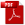Download as PDFCongruence properties of partitions
Proceedings of the London Mathematical Society, 2, XVIII, 1920, Records for 13 March 1919

In a paper published recently in the Proceedings of the Cambridge Philosophical Society1, I proved a number of arithmetical properties of $p(n)$, the number of unrestricted partitions of $n$, and in particular that $$p(5n+4)\equiv 0\pmod{5},$$ and $$p(7n+5)\equiv 0\pmod{7}.$$ Alternative proofs of these two theorems were found afterwards by Mr. H. B. C. Darling2.

I have since found another method which enables me to prove all these properties and a variety of others, of which the most striking is $$p(11n+6)\equiv 0\pmod{11}.$$ There are also corresponding properties in which the moduli are powers of 5, 7, or 11; thus $$p(25n+24)\equiv 0\pmod{25},$$ $$p(49n+19),\quad p(49n+33),\quad p(49n+40),\quad p(49n+47)\equiv 0\pmod{49},$$ $$p(121n+116)\equiv 0\pmod{121}.$$ It appears that there are no equally simple properties for any moduli involving primes other than these three.

The function $\tau (n)$ defined by the equation $$\sum_1^{\infty}\tau (n)x^n=x\{(1-x)(1-x^2)(1-x^3)\cdots\}^{24},$$ also possesses very remarkable arithmetical properties. Thus $$\tau (5n)\equiv 0\pmod{5},$$ $$\tau (7n),\quad \tau (7n+3),\quad \tau (7n+5),\quad \tau (7n+6)\equiv 0\pmod{7},$$ while $$\tau (23n+\nu )\equiv 0\pmod{23},$$ if $\nu$ is any one of the numbers $$5, 7, 10, 11, 14, 15, 17, 19, 20, 21, 22.$$

Endnotes

1Vol. XIX, 1919, pp. 207 –210 [No. 25 of this volume; see also No. 30].

2Ibid., pp. 217, 218.